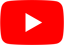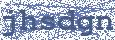Evan Finds The Third Room
Khruangbin

## KHRUANGBIN

Evan Finds The Third Room Lyrics

Yes, yes, yes, yes
Yes, this is the third room
Yes, this is the third room
Yes, this is the third room
Yes, this is the third room
Yes, this is the third room
Yes, this is the third room
Yes, this is the third room
Yes, this is the third room

Jump in the water
Jump in the water

Yes, yes, yes, yes
Yes, this is the third room
Yes, this is the third room
Yes, this is the third room
Yes, this is the third room

Jump in the water
Jump in the water
Jump in the water
Jump in the water

This is the third room
Yes, this is the third room
Yes, this is the third room
Yes, this is the third room

Press one
Press two
This is the third room
Press three
Press one
This is the third room
Press two
Press three
This is the third room
Hello, yes, this is the third room
If you'd like to say hello, press 1
Hello, hello
Press 2
Jump in the water
If you'd like to know if I'm alright, press 3, say, "You alright?"
This is the third room

Yes, jump in the water
Yes, this is the third room
Yes, this is the third room
Yes, this is the third roomHottest Lyrics with Videos
48e39091c971f44b264d022db648a493